Thank you for visiting nature.com. You are using a browser version with limited support for CSS. To obtain the best experience, we recommend you use a more up to date browser (or turn off compatibility mode in Internet Explorer). In the meantime, to ensure continued support, we are displaying the site without styles and JavaScript.

# Nonlinear spin-current enhancement enabled by spin-damping tuning

## Abstract

When a magnon, the quanta of a spin excitation, is created in a magnet, this quasiparticle can split into two magnons, which triggers an angular momentum flow from the lattice to the spin subsystem. Although this process is known to enhance spin-current emission at metal/magnetic insulator interfaces, the role of interacting magnons in spintronic devices is still not well-understood. Here, we show that the enhanced spin-current emission is enabled by spin-damping tuning triggered by the redistribution of magnons. This is evidenced by time-resolved measurements of magnon lifetimes using the inverse spin Hall effect. Furthermore, we demonstrate nonlinear enhancement of the spin conversion triggered by scattering processes that conserve the number of magnons, illustrating the crucial role of spin-damping tuning in the nonlinear spin-current emission. These findings provide a crucial piece of information for the development of nonlinear spin-based devices, promising important advances in insulator spintronics.

## Introduction

For more than half a century, the physics of spin dynamics has provided insight into the fundamental aspects of magnetism1,2,3,4. The collective excitations of localized spins coupled by the magnetic dipole and quantum exchange interactions are called spin waves or, in quantized form, magnons5. These quasiparticles, associated with the elementary magnetic excitation, are responsible for a flow of spin angular momentum, a spin current, in magnetic insulators, which are electrically inactive due to the frozen charge degrees of freedom, but magnetically active as a result of the interacting localized spins. In contrast to spin currents carried by conduction electrons in metals and semiconductors, which disappear within very short distances, spin currents in magnetic insulators persist over macroscopic distances owing to the substantially long lifetimes of the magnons relative to that of the conduction electron spins.

The recent discovery of spin-carrier conversion from magnons to conduction electrons through dynamical spin exchange coupling at metal/magnetic insulator interfaces sheds new light on the physics of nonlinear spin dynamics, leading to the combination of spintronics with interacting magnons. In a metal/magnetic insulator junction, the excitation of nonequilibrium magnons in the magnetic layer emits a spin current into the attached metal through spin pumping6,7,8,9,10,11,12,13,14,15,16. The spin-current emission has been found to be enhanced through nonlinear magnon interactions13,17. Although the physics behind the spin-current enhancement is still not clear, this illustrates the crucial role of nonlinear spin dynamics and interacting magnons in spintronic devices.

In this work, we demonstrate that the stabilized enhancement of the spin-current emission is enabled by spin-damping tuning, resulting from the redistribution of magnons due to the nonlinear magnon interactions. Of particular interest is the nonlinear three-magnon splitting, the splitting of one magnon into a pair of magnons (see Fig. 1a). Since each magnon carries spin angular momentum, in the exchange limit, it is natural to expect that the splitting directly enhances the spin-current emission. However, this picture is valid only for the time scale of the spin-lattice relaxation; the steady-state enhancement of the spin-current emission is governed by the spin damping. Our direct measurements of the spin damping demonstrate that the stabilized enhancement of the spin-current emission is enabled by the long lifetime of the dipole-exchange secondary magnons created at the inflection point of the dispersion curve, where the negative dipolar dispersion is compensated by the positive exchange dispersion. We demonstrate, furthermore, that the spin-current emission can be enhanced even in the absence of the magnon splitting; we found enhanced spin-current emission triggered by scattering processes that conserve the number of magnons. These findings demonstrate the crucial role of magnon lifetimes in spintronic devices, opening a way for controlling nonlinear spin-current emission through spin-damping tuning.

## Results

### Enhancement of spin-current emission

The nonlinear spin-current emission from a magnetic insulator is investigated by measuring the voltage generated through the inverse spin Hall effect (ISHE) in a Pt/Y3Fe5O12 (Pt/YIG) film, shown in Fig. 1b (details are outlined in Methods). Figure 1c shows the in-plane magnetic field, H, dependence of the microwave absorption intensity, P, measured by applying a 5 mW microwave with a fixed frequency of f0=5 GHz at T=250 K. Figure 1c shows that at H=HR=81.3 kA m−1, H fulfills the ferromagnetic resonance condition: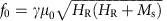, where γ is the gyromagnetic ratio and Ms is the saturation magnetization of the YIG layer. Around H=HR, we found voltage difference, V, between the ends of the Pt layer as shown in Fig. 1c. This provides evidence of spin-current emission from the YIG layer into the Pt layer; nonequilibrium magnons, excited by the magnetic resonance, emit a spin current into the Pt layer18,19, which is converted into an electric voltage by the ISHE in the Pt layer (see Fig. 1b)20,21,22.

The amplitude of the spin-current emission critically depends on the microwave excitation frequency, f0, because of the nonlinear dynamics of the interacting spins in the YIG layer. Figure 1d shows the field dependence of V(H)/Pabs, measured at T=250 K for various f0 values, where Pabs is the microwave absorption intensity (see Fig. 1c). Here V(H)/Pabs characterizes the conversion efficiency of the angular momentum created by the microwave field into the spin current carried by the conduction electrons in the Pt layer. Notably, V(H)/Pabs abruptly increases below f0=fc=3.4 GHz, while it is nearly constant for f0>3.5 GHz. To study the f0 dependence of V(H)/Pabs in detail, we plot κ(f0, T)≡VISHE(f0, T)/Pabs(f0, T) at different f0 values in Fig. 1e, where VISHE is the magnitude of the electric voltage. Figure 1e shows that κ(f0, T=250 K) for f0>3.5 GHz is well-reproduced with a calculation based on the linear spin pumping model23 (see the red line in Fig. 1e), demonstrating that the enhancement of κ(f0, T=250 K) at low f0 originates from the nonlinearity of the spin dynamics in the YIG layer: the three-magnon splitting17. Here the microwave excitation power Pin=5 mW is about 3 orders of magnitude larger than the threshold power of the three-magnon splitting5,17.

### Spin-current emission at various temperatures

Importantly, the conversion efficiency κ is enhanced at low temperatures. Figure 2a shows the f0 dependence of the conversion efficiency κ(f0, T)=VISHE(f0, T)/Pabs(f0, T) at different temperatures T, where κ(f0, T) is divided by κ(f0=5 GHz, T) to omit the temperature dependent parameters relevant to the conversion efficiency from the spin current to the electric voltage such as the spin Hall angle, electrical conductivity and spin diffusion length in the Pt layer (see the inset to Fig. 2a). Notably, κ(f0, T) clearly increases with decreasing temperature under the three-magnon splitting (see also Fig. 2b). Figure 2a also shows that the cut-off frequency, fc, below which κ(f0, T) increases abruptly, increases with decreasing temperature (see Fig. 2c).

We first discuss the T dependence of fc, which further supports that the three-magnon splitting is responsible for the enhancement of the spin-current emission increased at low temperatures. The three-magnon splitting creates a pair of magnons with opposite wavevectors and frequency f0/2 from the uniform magnon with a frequency of f0 (see Fig. 1a), following the energy and momentum conservation laws. Thus, the splitting process is only allowed for f0/2>fmin, where fmin is the minimum frequency of the magnon dispersion as shown in Fig. 1a. fmin for the thin YIG film used in the present study can be obtained from the dipole-exchange magnon dispersion. The lowest branch of the magnon dispersion is expressed as24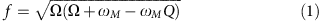where Ω=ωH+ωM(D/μ0Ms)K2, ωH=γμ0H, ωM=γμ0Ms, K2=λ2+k2=(π/L)2+k2, and Q=(k/K)2[1+(λ/K)2(2/kL) [1+exp(−kL)]]. D is the exchange interaction constant, L is the thickness of the YIG layer and k is the wavenumber of the magnons. To obtain the exact condition f0/2>fmin for the Pt/YIG film, a detailed calculation using equation (1) is necessary as discussed below. Before discussing in detail the condition for the three-magnon splitting based on the exact magnon dispersion, we first neglect the surface dipolar interactions in equation (1) for simplicity. fmin under this approximation can be obtained in the limit of L→∞ as fminγμ0H. This relation with the resonance frequencyshows that the condition f0/2>fmin for the three-magnon splitting is satisfied when f0<(2/3) γμ0Ms. This predicts that fc increases with Ms due to the change in the magnon dispersion. We estimated Ms of the Pt/YIG film from the resonance field HR using the Kittel formula (see Fig. 3a) as shown in Fig. 3b. Using this result, we plot the relationship between fc and Ms in Fig. 3c, which is consistent with the above discussion—fc increases with Ms. Thus, the observed change in fc can be attributed to the change in the magnon dispersion, caused by the temperature variation of Ms in the Pt/YIG film.

The experimentally measured temperature dependences of fc and Ms shown in Figs 2c and 3b illustrate the exact magnon dispersion of the Pt/YIG film at each temperature using equation (1). When the external magnetic field H is varied, the magnon dispersion is shifted in frequency space and the relationship between the frequencies f0/2 and fmin also changes. Figure 4a shows a plot of the H dependences of f0/2 and fmin at T=100 and 300 K, where f0/2 was calculated using the Kittel formula with the experimentally obtained Ms. fmin was plotted by finding the minimum frequency of the magnon dispersion using equation(1). The crossing points of f0/2 and fmin correspond to the cut-off frequency fc, where fmin and the crossing point can be varied by changing the exchange interaction constant D; D for which the crossing point reproduces the measured fc value at different temperatures is plotted in the inset to Fig. 4b. The experimentally obtained D is almost temperature independent, consistent with the literature25. Using the values of Ms and D, we plot the lowest branch of the magnon dispersion at the cut-off frequency, f0=fc=2fmin, for different temperatures in Fig. 4b. Figure 4b shows that the shape of the lowest branch of the magnon dispersion changes slightly by changing temperature, which is the origin of the temperature dependence of the cut-off frequency.

## Discussion

The observed enhancement of the spin-current emission is enabled by spin-damping tuning triggered by the redistribution of magnons due to the nonlinear magnon scattering. Here note that the steady-state spin-current emission from the magnetic insulator is stabilized by balancing the creation and decay of the magnons; the steady-state angular momentum stored in the spin system is governed by the magnon damping. Assuming that the spin current emitted from the magnetic insulator is proportional to the total number of nonequilibrium magnons26,27,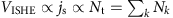and using the energy balance relation Pabs=∑kωkηkNk, we find that the conversion efficiency κ=VISHE/Pabs is proportional to the spin-lattice relaxation time τ of the pumped system: κτ. The relaxation rate 1/τ is given by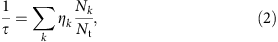where Nk and ηk are the number and the damping of nonequilibrium magnons with the wavenumber k, respectively, and Nt=∑kNk is the total number of nonequilibrium magnons in the system. This simple model provides a general picture of the nonlinear spin-current emission in the presence of magnon scatterings; the above model indicates that the spin-current emission can be enhanced through the magnon redistribution in a system with η0>ηq, where η0 and ηq are the damping of the uniform and secondary magnons, respectively. In this system, the magnon redistribution triggered by magnon interactions destroys the magnons with the large-damping η0 and creates the magnons with the small-damping ηq, changing the relative weight Nk/Nt in equation (2) and decreasing the dissipation rate 1/τ of the angular momentum from the spin system to the lattice. This spin-damping tuning, triggered by the magnon redistribution, increases the steady-state angular momentum stored in the spin system, giving rise to the stabilized enhancement of the spin-current emission.

The spin damping 1/τ can be measured directly by monitoring the temporal evolution of the spin-current emission using the ISHE, which provides direct evidence that the spin damping plays a key role in the spin-current enhancement. Figure 5a shows the temporal evolution of the electric voltage due to the ISHE in the absence of the three-magnon splitting: f0=8 GHz>fc. The temporal profile of the ISHE voltage, VR(t), at the resonance field, H=HR, was measured by applying a microwave pulse that was switched off at t=tMW. Figure 5a shows that VR(t) decays exponentially after switching off the microwaves; the spin-current emission decreases with time due to the dissipation of the angular momentum from the spin system to the lattice in the YIG layer28. The spin-current decay was also observed at f0=2.8 GHz<fc as shown in Fig. 5b, where the dipole-exchange magnons are created by the three-magnon splitting. For these results, we extracted the relaxation time, τ, by fitting the voltage with VR(t)/VR(t=tMW−500 ns)=exp(−2t/τ) for t>tMW. The extracted relaxation time τ for different f0 at T=300 K is shown in Fig. 5c. Figure 5c shows that τ is almost constant for f0>fc. In contrast, τ increases with decreasing the excitation frequency for f0<fc, reminiscent of the f0 dependence of κ shown in Fig. 2a. These results, therefore, demonstrate the direct relationship between the spin damping and the conversion efficiency in the nonlinear spin-current emission.

The observed spin-current enhancement and spin-damping tuning is realized by the small damping and long-lived nature of the dipole-exchange secondary magnons created by the three-magnon splitting. When f0<fc, the three-magnon splitting creates the dipole-exchange secondary magnons with wavevectors of kq from the k=0 uniform magnons near the bottom of the magnon dispersion as shown in Fig. 1a. Although the damping of the uniform magnon is dominated by the elastic two-magnon scattering due to nonuniformities, where a magnon is destroyed and another magnon at the same frequency is created29, the two-magnon scattering can be suppressed for the dipole-exchange secondary magnons created by the three-magnon splitting. For the secondary magnons, because of the nearly zero group velocity due to the competition of the magnetic dipole and quantum exchange interactions, the scattering events due to nonuniformities are suppressed, resulting in the long lifetime, or the small damping. In the presence of the three-magnon splitting, the dynamics of these uniform and secondary magnons are modelled as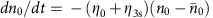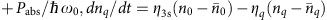and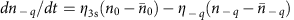, because short wavelength magnons do not couple to the microwave field. nk(k=0, ±q) is the number of the magnons with the wavenumber k and the damping ηk.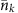is the thermodynamic equilibrium value of nk. Pabs is the absorbed microwave power and ω0=2πf0. Here for simplicity, we assume that the damping due to the three-magnon splitting as η3s3s(nq+nq), where Γ3s is the splitting strength.5,30 Notably, the splitting strength Γ3s increases with the decreasing excitation frequency f0, because the number of the degenerate states at f0/2 available for the splitting increases by decreasing f0 (see also the schematic illustrations in Fig. 5c)30. Thus, by decreasing f0 below the cut-off frequency, the relative number of the small-damping dipole-exchange secondary magnons increases and that of the large-damping uniform magnons decreases (see also equation (2)), resulting in the long lifetime τ of the spin system as demonstrated in Fig. 5c. The effect of the creation of an additional magnon through the splitting on κ becomes evident in a strong excitation condition. For Γ3s≠0, from the above rate equations, the number of the nonequilibrium uniform magnons can be expressed as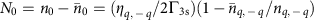, where nq,−qnq=nq and ηq,−qηq=ηq. In the limit of strong excitation, that is,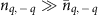, the number of the nonequilibrium uniform magnons is limited to N0ηq,−q/2Γ3s. Thus, in this condition,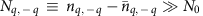and Nt2Nq,−q, resulting in κ3s=VISHE/Pabs2/ηq,−q because of the approximated energy balance relation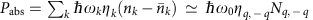. The factor 2 arises from the fact that the three-magnon splitting creates two magnons from one magnon. Although the three-magnon splitting triggers the angular momentum flow from the lattice, the creation of the secondary magnons also affects the inherent dissipation of the angular momentum from the magnons to the lattice; when the redistribution of magnons creates secondary magnons with exceptionally short lifetimes, this redistribution process enhances the inherent dissipation of the angular momentum of the spin system or decreases τ of the system, masking the angular momentum flow from the lattice triggered by the nonlinear magnon interaction. Thus, in the steady state, it is nontrivial whether the magnon splitting increases or decreases the nonequilibrium angular momentum stored in the spin system. Since in the absence of the splitting, Γ3s=0, the conversion efficiency is expressed as κlinear1/η0, the spin-current emission can be enhanced by the three-magnon splitting when the damping of the uniform and secondary magnons satisfies κ3s/κlinear=2η0/ηq,−q>1; the creation of long-lived magnons with a damping of ηq,−q(<2η0) through the nonlinear magnon scattering is responsible for the enhancement of the spin-current emission.

The temperature dependence of the conversion efficiency κ shown in Fig. 2b further demonstrates that the spin-current emission is governed by the spin damping of the system. Figure 5a shows that the decay time of VR(t) is almost independent of temperature when f0=8 GHz>fc, that is, in the absence of the three-magnon splitting. In contrast, the voltage decay time varies by changing the temperature at f0=2.8 GHz<fc, as shown in Fig. 5b. The temperature dependence of the decay time, τ, is summarized in Fig. 5d. This result shows that the magnon relaxation time τ increases with decreasing temperature under the three-magnon splitting, consistent with the experimentally obtained temperature dependence of κ shown in Fig. 2b. At the fixed excitation frequency f0, the number of the available states at f0/2 for the splitting increases with decreasing temperature (see Fig. 5d). This indicates that the relative number of the small-damping secondary magnons increases with decreasing temperature, which reduces the damping of the spin system through the splitting. The damping of the secondary magnon itself is also expected to be reduced with decreasing temperature, since the damping of the dipole-exchange magnon is dominated by the three-particle processes, which is almost proportional to temperature29,31. Therefore, by decreasing temperature, both the splitting strength and the damping of the secondary magnons tend to increase the relaxation time of the spin system, giving rise to the experimentally observed longer τ at low temperatures.

The direct measurements of the spin damping at different frequencies and temperatures shown in Fig. 5 provide evidence that the creation of the long-lived secondary dipole-exchange magnons through the redistribution is essential for the enhancement of the spin-current emission. This picture is further evidenced by microwave excitation power Pin dependence of the conversion efficiency κ(Pin)=VISHE(Pin)/Pabs(Pin) for the Pt/YIG film shown in Fig. 6a. The applied microwave excitation frequency was f0=7.6 GHz>fc, that is, the three-magnon splitting is prohibited in this measurement. For Pin<Pth, the conversion efficiency is constant, consistent with the spin-current emission in the linear regime32; the emitted spin current is proportional to Pin. However, κ(Pin) clearly increases by increasing Pin for Pin>Pth, demonstrating the enhancement of the spin conversion efficiency without the splitting of a pumped magnon, or without breaking the angular momentum conservation of the spin subsystem. Notably, κ(Pin) shows a clear threshold power Pth, indicating that the four-magnon scattering33,34, where two magnons are created with the annihilation of two other magnons, is responsible for the enhanced spin-current emission.

The enhanced spin-current emission induced by the scattering process that conserve the number of magnons, that is, the four-magnon scattering, demonstrates the general picture of the role of the magnon interactions in the nonlinear spin-current emission: the spin-damping tuning triggered by the magnon redistribution. In the absence of the redistribution of magnons, the angular momentum relaxation rate from the spin system to the lattice is 1/τη0 because of NtN0. Although the four-magnon scattering creates dipole-exchange magnons at the excitation frequency f0 not at f0/2, the lifetime of these magnons can be longer than that of the uniform magnon because of the small group velocity35. Thus, even in the absence of the three-magnon splitting, the four-magnon scattering can decrease the relaxation rate of the spin system, 1/τ, through the annihilation of the uniform magnons with large η0 and creation of dipole-exchange magnons with small ηq, resulting in the steady-state enhancement of the angular momentum stored in the spin system or the enhanced spin-current emission shown in Fig. 6a. This is further supported by a rate equation approach for the four-magnon scattering33,36,37: dN0/dt=−[η0+ηspf(Pin)]N0+Pabs/ω0 and d(NtN0)/dt=−ηq(NtN0)+ηspf(Pin)N0, where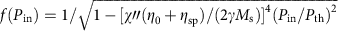. ηsp is the decay constant of the uniform precession to degenerate magnons at f0 due to scattering from sample inhomogeneities. To keep the discussion simple, we define ηq as the average decay rate to the thermodynamic equilibrium of the degenerate secondary magnons. Pth is the threshold power for the four-magnon scattering and χ″ is the imaginary part of the susceptibility. Pabs=ω0χh2 and h is the applied microwave field strength. The steady-state solution of the above rate equation gives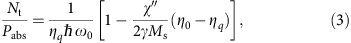where κ(Pin)Nt/Pabs and χ″=(2γMs)/(η0+ηspf(Pin)). Figure 6b shows the conversion efficiency κ(Pin) calculated using equation (3) for ηq/η0=0.75 (the red curve) and ηq/η0=1.25 (the black curve) with the assumption of VISHENt. In a system with ηq/η0=1.25, κ(Pin) decreases with Pin for Pin>Pth, since the scattering process increases the relative number of the large-damping magnons and increases the dissipation rate of the angular momentum stored in the spin system (see equation (2)). In contrast, in a system with ηq/η0=0.75, the four-magnon scattering increases the relative number of the small-damping magnons and decreases the damping of the spin system, resulting in the enhanced spin-current emission, which is consistent with the experimental observation shown in Fig. 6a.

The observed enhancement of the spin-current emission demonstrates the crucial role of the spin damping affected by nonlinear magnon interactions in spintronic devices. The damping of the spin system can be directly quantified using the time-resolved measurement of the spin-current emission under various conditions, providing a way for further development of the physics of nonlinear spin conversion. These findings shed new light on nonlinear spin dynamics, promising important advances in spintronics with interacting magnons.

## Methods

### Sample preparation

The sample used in this study is a Pt/YIG film. A single-crystal YIG (111) film (3 × 5 mm2) with a thickness of 5 μm was grown on a Gd3Ga5O12 (111) substrate by liquid phase epitaxy. A 10-nm-thick Pt layer was sputtered in an Ar atmosphere on top of the film and two electrodes were attached to the edges of the Pt layer for voltage measurements.

### ISHE voltage and microwave absorption measurements

The Pt/YIG film was placed at the centre of a coplanar waveguide, where a microwave signal with a frequency of f0 was applied to the input of the signal line. The signal line of the coplanar waveguide is 500 μm wide and the gaps between the signal line and the ground lines are designed to match the characteristic impedance of 50 Ω. An in-plane external magnetic field was applied parallel to the signal line, such that the static magnetic field is perpendicular to the dynamic magnetic field produced by the microwave current. The microwave power absorbed by the sample Pabs was measured by monitoring the transmitted output power.

How to cite this article: Sakimura, H. et al. Nonlinear spin-current enhancement enabled by spin-damping tuning. Nat. Commun. 5:5730 doi: 10.1038/ncomms6730 (2014).

## References

1. 1

Serga, A. A., Chumak, A. V. & Hillebrands, B. YIG magnonics. J. Phys. D 43, 264002 (2010).

2. 2

Lenk, B., Ulrichs, H., Garbs, F. & Munzenberg, M. The building blocks of magnonics. Phys. Rep. 507, 107–136 (2011).

3. 3

Patton, C. E. Magnetic excitations in solids. Phys. Rep. 103, 251–315 (1984).

4. 4

L'vov, V. S. Wave Turbulence Under Parametric Excitation Springer-Verlag (1994).

5. 5

Gurevich, A. G. & Melkov, G. A. Magnetization Oscillations and Waves CRC Press (1996).

6. 6

Kajiwara, Y. et al. Transmission of electrical signals by spin-wave interconversion in a magnetic insulator. Nature 464, 262–266 (2010).

7. 7

Sun, Y. et al. Damping in yttrium iron garnet nanoscale films capped by platinum. Phys. Rev. Lett. 111, 106601 (2013).

8. 8

Heinrich, B. et al. Spin pumping at the magnetic insulator (YIG)/normal metal (Au) interfaces. Phys. Rev. Lett. 107, 066604 (2011).

9. 9

Hahn, C. et al. Comparative measurements of inverse spin Hall effects and magnetoresistance in YIG/Pt and YIG/Ta. Phys. Rev. B 87, 174417 (2013).

10. 10

Weiler, M. et al. Experimental test of the spin mixing interface conductivity concept. Phys. Rev. Lett. 111, 176601 (2013).

11. 11

Rezende, S. M., Rodriguez-Suarez, R. L. & Azevedo, A. Magnetic relaxation due to spin pumping in thick ferromagnetic films in contact with normal metals. Phys. Rev. B 88, 014404 (2013).

12. 12

d'Allivy Kelly, O. et al. Inverse spin Hall effect in nanometer-thick yttrium iron garnet/Pt system. Appl. Phys. Lett. 103, 082408 (2013).

13. 13

Castel, V., Vlietstra, N., van Wees, B. J. & Youssef, J. B. Frequency and power dependence of spin-current emission by spin pumping in a thin-film YIG/Pt system. Phys. Rev. B 86, 134419 (2012).

14. 14

Sandweg, C. W. et al. Spin pumping by parametrically excited exchange magnons. Phys. Rev. Lett. 106, 216601 (2011).

15. 15

Hahn, C. et al. Detection of microwave spin pumping using the inverse spin Hall effect. Phys. Rev. Lett. 111, 217204 (2013).

16. 16

Jiao, H. & Bauer, G. E. W. Spin backflow and ac voltage generation by spin pumping and the inverse spin Hall effect. Phys. Rev. Lett. 110, 217602 (2013).

17. 17

Kurebayashi, H. et al. Controlled enhancement of spin-current emission by three-magnon splitting. Nat. Mater. 10, 660–664 (2011).

18. 18

Tserkovnyak, Y., Brataas, A. & Bauer, G. E. W. Enhanced Gilbert damping in thin ferromagnetic films. Phys. Rev. Lett. 88, 117601 (2002).

19. 19

Brataas, A., Tserkovnyak, Y., Bauer, G. E. W. & Halperin, B. I. Spin battery operated by ferromagnetic resonance. Phys. Rev. B 66, 060404(R) (2002).

20. 20

Saitoh, E., Ueda, M., Miyajima, H. & Tatara, G. Conversion of spin current into charge current at room temperature: Inverse spin-Hall effect. Appl. Phys. Lett. 88, 182509 (2006).

21. 21

Kimura, T., Otani, Y., Sato, T., Takahashi, S. & Maekawa, S. Room-temperature reversible spin Hall effect. Phys. Rev. Lett. 98, 156601 (2007).

22. 22

Valenzuela, S. O. & Tinkham, M. Direct electronic measurement of the spin Hall effect. Nature 442, 176–179 (2006).

23. 23

Harii, K. et al. Frequency dependence of spin pumping in Pt/Y3Fe5O12 film. J. Appl. Phys. 109, 116105 (2011).

24. 24

Kalinikos, B. Excitation of propagating spin waves in ferromagnetic films. Microw., Opt. Antenna IEE Proc. H 127, 4–10 (1980).

25. 25

LeCraw, R. C. & Walker, L. R. Temperature dependence of the spin-wave spectrum of yttrium iron garnet. J. Appl. Phys. 32, S167–S168 (1961).

26. 26

Chumak, A. V. et al. Direct detection of magnon spin transport by the inverse spin Hall effect. Appl. Phys. Lett. 100, 082405 (2012).

27. 27

Kurebayashi, H. et al. Spin pumping by parametrically excited short-wavelength spin waves. Appl. Phys. Lett. 99, 162502 (2011).

28. 28

Jungfleisch, M. B. et al. Temporal evolution of inverse spin Hall effect voltage in a magnetic insulator-nonmagnetic metal structure. Appl. Phys. Lett. 99, 182512 (2011).

29. 29

Sparks, M. Ferromagnetic-Relaxation Theory McGraw-Hill (1964).

30. 30

Chernyshev, A. L. Field dependence of magnon decay in yttrium iron garnet thin films. Phys. Rev. B 86, 060401 (2012).

31. 31

Kolokolov, I., L'vov, V. & Cherepanov, V. Magnon interaction and relaxation in yttrium iron garnet, a twenty-sublattice ferromagnet. Sov. Phys. J. Exp. Theor. Phys. 59, 1131–1139 (1984).

32. 32

Ando, K. et al. Inverse spin-Hall effect induced by spin pumping in metallic system. J. Appl. Phys. 109, 103913 (2011).

33. 33

Suhl, H. The theory of ferromagnetic resonance at high signal powers. J. Phys. Chem. Solids 1, 209–227 (1957).

34. 34

Schultheiss, H., Vogt, K. & Hillebrands, B. Direct observation of nonlinear four-magnon scattering in spin-wave microconduits. Phys. Rev. B 86, 054414 (2012).

35. 35

Melkov, G. A., Vasyuchka, V. I., Kobljanskyj, Y. V. & Slavin, A. N. Wave-front reversal in a medium with inhomogeneities and an anisotropic wave spectrum. Phys. Rev. B 70, 224407 (2004).

36. 36

Suhl, H. Note on the saturation of the main resonance in ferromagnetics. J. Appl. Phys. 30, 1961–1964 (1959).

37. 37

de Loubens, G., Naletov, V. V. & Klein, O. Reduction of the spin-wave damping induced by nonlinear effects. Phys. Rev. B 71, 180411 (2005).

## Acknowledgements

We thank A.L. Chernyshev, H. Kurebayashi, Y. Kajiwara and M.B. Jungfleisch for valuable discussions. This work was supported by PRESTO-JST ‘Innovative nano-electronics through interdisciplinary collaboration among material, device and system layers,‘JSPS KAKENHI Grant Numbers 26220604, 26103004, 26600078, the Mitsubishi Foundation, the Asahi Glass Foundation, the Noguchi Institute, the Murata Science Foundation and the Cabinet Office, Government of Japan through its ‘Funding Programme for Next Generation World-Leading Researchers’.

## Author information

Authors

### Contributions

K.A. designed the experiments, developed the explanation and wrote the manuscript. H.S. and T.T. collected and analyzed the data. All authors discussed the results and reviewed the manuscript.

### Corresponding author

Correspondence to Kazuya Ando.

## Ethics declarations

### Competing interests

The authors declare no competing financial interests.

## Rights and permissions

Reprints and Permissions

• ### Generation of megahertz-band spin currents using nonlinear spin pumping

• Shingo Watanabe
• Daichi Hirobe
• Eiji Saitoh

Scientific Reports (2017)

• ### Yttrium Iron Garnet Thin Films with Very Low Damping Obtained by Recrystallization of Amorphous Material

• Christoph Hauser
• Tim Richter
• Georg Schmidt

Scientific Reports (2016)

• ### Spin-current emission governed by nonlinear spin dynamics

• Takaharu Tashiro
• Saki Matsuura
• Kazuya Ando

Scientific Reports (2015)